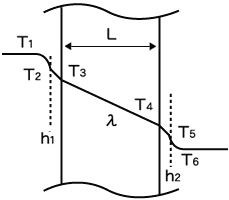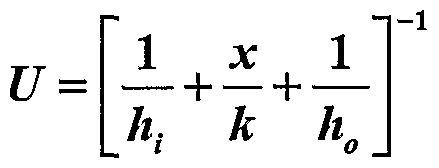# Pharma Engineering

For Engineer By An Engineer

• ## Saturday, 14 May 2016

Hello guys........Hope all are enjoying the start of these weekend !!

Today i wanna add a full stop for many peoples fantasies of 'U' value calculation, the most common line that i heard from many engineers was Consider U as 150 for Reactors and 450 for Condensers, in return if you ask them what are the units for those 150 and 450, many of them will take some strategic time out as in IPL.

An Engineer is the one who holds the ability to deal any of the situation with a smart work, but not the one who follow the Thumb blindly, i'm not criticizing the Thumb, as many cases i too prefer the Thumb rules in practical cases but my intention is An Engineer should be able to derive the U value if needed.

And for that we need to know some basics, before going into topic.

What are Heat Transfer Co-Efficient?

Heat transfer coefficient is a quantitative characteristic of convective heat transfer between a fluid medium (a fluid) and the surface (wall) flowed over by the fluid.

What is Overall Heat Transfer Co-efficient?

Overall Heat Transfer Co-efficient is the combination of the possible Individual Heat Transfer Co-efficient, which are due to the following Heat Transfer modes,
1. Rate of convective heat transfer from Fluid to Medium Wall, and
2. Conductive Heat Transfer from Medium Walls one end to other end,
3. Convective Heat Transfer from Medium wall to inside Fluid.Credits: TLV.com

What is Thermal Conductivity?

Thermal Conductivity of a material characterizes the Rate of Heat Transfer of a material, whether the heat is flowing in or out.

The thermal conductivity - k - for some typical materials (varies with temperature)
• Polypropylene PP : 0.1 - 0.22 W/mK
• Stainless steel : 16 - 24 W/mK
• Aluminum : 205 - 250 W/mK
• Glass lining :  1.2 W/mK
What are the units of Overall Heat Transfer Co-efficient and How are they derived?

British Units for U are Btu/(hr-ft2°F) , SI units for U are Watts/Sq.m .°K or KCal/Sq.m .sec.°K

They are basically derived from the formula Q = U x A x LMTD,

where Q holds the units Watts , A - Sq.m, LMTD - °K or °C.

So, that's how U units were derived.Credits: engineersedge.com

What will be the Trend of Convective Heat Transfer Co- efficients?

The Convective Heat Transfer Trend will be as follows:

 Fluid Convective heat transfer coefficient (h) Water about 1000 W/(m2°C) Hot Water 1000 – 6000 W/(m2)°C Steam 6000 – 15000 W/(m2°C)

The convection heat transfer coefficient - h - depends on
1. The type of fluid - gas or liquid,
2. The flow properties such as velocity,
3. Other flow and temperature dependent properties.
Convective heat transfer coefficient for some common fluids:
• Air - 10 to 100 W/m2K
• Water - 500 to 10 000 W/m2K
So, I think right now you acquired some courage to drive the fantasy of  'U' value calculation from your mind. And, i'll start the main show now.

U value Calculation:

For calculation of U value we need some basic data regarding the Jacket fluid properties, Vessel Mass properties, Jacket properties, Equipment Physical data  etc.

General Data includes: Vessel Dia, Vessel MOC, Vessel wall thickness.

For Calculating Overall Heat Transfer Coefficient we need Individual Heat Transfer Co-efficients used in the following co-relation.

1 / U = ( 1 / hj ) + ( Fj ) + ( X/K ) + Fv + ( 1/hi )
The hj, hi need to be calculated primarily,

Jacket Side Heat Transfer Co-efficient Calculation:

For Calculation of  hj , we need to know Pipe coil ID, Flowrate in pipe, Fouling factor Fj, Fluid properties[Density, Viscosity, Specific heat, Conductivity],

With an example i'll illustrate here,

Let pipe ID is 2", Flowrate(Q) is 10 Cu.m/hr, Fouling factor(Fj) = 0.0002 h.m2.°C/Kcal,

Density (rho) 1000 Kg/Cu.m, Viscosity(μ) 0.01 cP, Specific heat (Cp) 0.9 KCal/Kg.°C, Conductivity(k) 0.2 KCal/m.h.°C,
Viscosity @ wall is w) = 0.01 cP

Velocity, V = Q/A = 2.7410 m/sec
Reynold's number, Nre = D x V x rho / μ =17638677,
Prandtl's number, Npr = Cp x μ / k = 0.2093

using the below co-relation ,

hj x D / K = 0.023 x ( Nre ^ 0.8 ) x ( Npr ^ 0.33 ) x (( μ/μw)^0.14)

hj = 27202.81 Kcal/h.m2.°C

Vessel Side Heat Transfer Co-efficient Calculation:

For this calculation we need to know the inside fluid properties, which includes Density, Viscosity, Conductivity, Specific Heat, Viscosity @ wall.

Within an example i'll illustrate here,

Agitator RPM 36, Agitator Dia 36", Density (rho) 900 Kg/Cu.m, Viscosity(μ) 2cP, Specific Heat(Cp) 1KCal/Kg.°C, Conductivity (k) 0.5 KCal/m.h.°C, Viscosity @wall (μw) 1 cP, Fouling factor(Fv) 0.0002 h.m2.°C/Kcal,

Reynold's number, Nre = N x D^2 x rho / μ = 313548,
Prandtl's number, Npr = Cp x μ / k = 14.4

Now, Individual Heat Transfer Co-efficient hi is Calculated by following co-relation,

hi x D / K = 0.53 x (Nre ^0.66) x ( Npr ^ 0.33) x (( μ/μw)^0.24)

hi = 1441.16 Kcal/h.m2.°C

So, Now our task came to an end,

U value Calculation,

1 / U = 1/hj + Fj + X/K + Fv + 1/hi

1 / U = `1/27202.8 + 0.0002 + 0.0007 + 0.0002 + 1/1441.2`

U = 537.019 Kcal/h.m2.°C

Actually one more thing i should have been included in the basic "What are the factor do the  U value depend?", anyway by the end of this tutorial you should have learned this,

Getting mails asking me to demonstrate with some example data, and that's why today i've illustrated the formulae with data,

If you understood clearly then say cheers, or any queries, feel free to contact me, M happy to help,

You May Also Like:Hi! I am Ajay Kumar Kalva, Currently serving as the CEO of this site, a tech geek by passion, and a chemical process engineer by profession, i'm interested in writing articles regarding technology, hacking and pharma technology.

1.Cheerz AJ 💜💜💜

1.2.Table 1. Typical overall coefficients for jacketed glass lined steel vessels
Duty U (W m−2K−1)
Distillation/Evaporation 350
Heating 310
Cooling 200
Cooling (chilled service) 100
Table 2. Typical overall coefficients for jacketed carbon and stainless steel vessels
Duty U (W m−2K−1)
Heating 400
Cooling 350
Cooling (chilled service) 150
A typical overall coefficient for a well designed coil would be 400 to 600 Wm2K−1.

1.These are Thumb and we can't strongly depend on these all,

3.This comment has been removed by the author.

4.Aj.. In batch reactor heating/ cooling,
Isothermal& Nonisothermal medium relates to.. ????

1.Sorry, can't get what you are asking...!! Query need to be some more clear

5.What is HVAC system?,explain it with diagram.

1.HVAC means Heating, ventilation and air conditioning systems, i'll explain it in my next post.

Regards,
AJAY KUMAR

6.How will you calculate Reynolds no.for flow reactor?

1.http://www.nuclear-power.net/nuclear-engineering/fluid-dynamics/reynolds-number/reynolds-number-for-pipe-flow/

if needed i'll explain that in a special post

Regards,
AJAY KUMAR

2.If I have 91 tr chiller -20°C,to cool the 30kl methonal,how much time required to bring the methanol to 30°C to -20°C

3.Dear Ganesan,

3.41 hours, i've replied back to your previous comment, check it for calculation.

Regards,
AJAY K

7.Dear sir ,

I have 91 tr chiller at -20°C,flow is about 65m3/hr,fluid used is methanol brine( 33% by weight).

And my brine tank capacity is 50kl,brine is 30 kl.

How much time required to bring the methanol brine from 30°C to -20°C.

Kindly explain little deeper because I am very new in this field

2.This may be very basic question.

I have 1 Lt water at 70°C,and another 3 litre at 20°C
If mix the both water, what is my output temperature

1.Dear Ganeshan,
1 Lt of water at 70°C and 3 Lt at 20°C,
1 x 1 x 1 x (70-X) = 3 x 1 x 1 x (X-20),
Solve for X, x in °C,
Formula used: M x Cp x dT.

1st question solution:

91 x 3024 = 30 x 1000 x 0.92 x 0.68 x (30 + 20 ) / T,
T in hrs.
Formula used: TR = [ M x Cp x dT / 3024 ]
Solve for T. thats' it

Sorry for the late resposnse.

regards,
AJAY K

8.Dear sir,
Thank you for your quick response

Could you explain little clearly because I am new to the field and also learner.

Cp=1 kcal/kgk(for water)
Delta T=(70-X)
M=? how you put mass flow rate

How to find out the time.I don't know

M=30*1000
Just tell the units your using
In final step how 'T' is come at bottom

1.Dear Ganesan,

1. M is nothing but amount of liquid that we would like to heat in time 't', so i've considered it as 3, and x 1 is the specific gravity of water to convert it into mass from volume, i.e., from Liters to Kg's.
2. i've taken 30 x 1000 becoz 30 is in KL and density of brine is around 0.92, and i've multiplied with 1000 coz to convert it into L.

Still any queries feel free to message / comment.

Regards,
AJAY K

9.How to find heat transfer coefficient for utility flow through conventional jacket?

1.Dear Natasha,

if its a conventional jacket (i.e., annular space jacket), then the volume occupied shall be calculated as overall volume minus central vessel volume, and the heat transfer area shall be the lateral surface area of the inner cylinder which shall be calculated based on the outer dia of the cylinder.

Regards,
AJAY K

10.Also, shouldn't we find overall heat transfer coefficient by:

1/U = 1/(hiAi) + 1/(hoAo) + x/k
Then we find Uo or Ui based on outer or inner surface area.

1.Dear Natasha,

As per my knowledge there wont be Uo & Ui, considering hi, ho we'll calculate U that will be applicable for heat load calculations, if needed you can add the fouling factors also as mentioned in above post.

Hope you received it, if any queries feel free to comment/contact.

Regards,
AJAY K

2.If I have calculate tubular heat outlet of products and cold water outlets
I know only inlet temperature of both and flow rate of products and HW.

11.How to find heat transfer coeficient of dimple jacket & limpet coil??

1.Hey....,

For this query, i can only speak upto my knowledge,
As dimple jackets are mostly used for steam application, the overall heat transfer coefficient can be considered on higher side, it may vary upto 400-500 KCal/hr.Sq.m.C,
If its a limpet coil we can estimate upto 250-350 KCal/hr.Sq.m.C,
If steam is used as utility for limpet coil then it may go upto 400 KCal/hr.Sq.m.C.

Best Regards,
AJAY K

12.Plz explain X/K

1.Dear Mangesh,

X/K is the ration of thickness of the vessel to the thermal conductivity of the MOC, Holds the inverse units of heat transfer coefficient.

Best Regards,
AJAY K

13.Can you share the excel sheet for this work up.

14.Few points :
1. The constant of Sieder Tate constant would be 0.027 instead of 0.023 during hj calculation;

2. I think we should use hydraulic mean dia of the pipe for calculations;

3. And ensure SI units conversion for calculations for parameters like viscosity

1.Hiii,

Thats dittus boelter not sieder tate,

Hydraulic mean dia can be used if the flow is in non-circular tubes, as the flow will be circular i've used this,

Yeah, might be the conversions are slightly confusing, but they are fine, from next time i'll go with SI units.

Next time, pl comment with your goodname.

Regards,
AJAY KHi! I am Ajay Kumar Kalva, Currently serving as the CEO of this site, a tech geek by passion, and a chemical process engineer by profession, i'm interested in writing articles regarding technology, hacking and pharma technology.